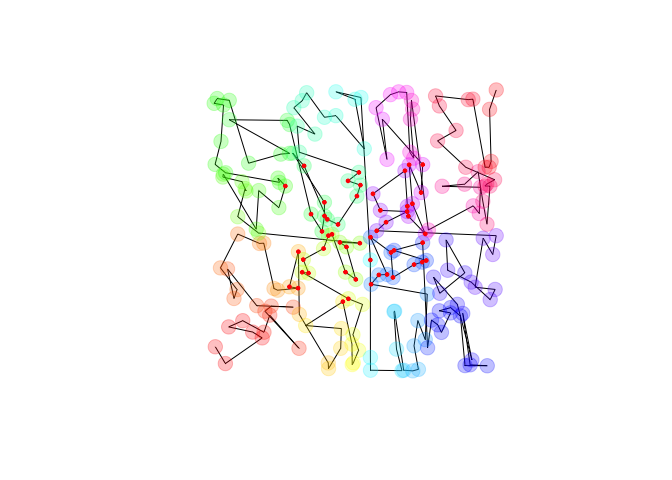# kdtools

The kdtools package exports a C++ header implementing sorting and searching on ranges of tuple-like objects without using trees. It is based on a kd-tree-like recursive sorting algorithm. Once sorted, one can perform a range- or nearest-neighbor- query. More details are here. Methods and benchmarks are here.

``````library(kdtools)
x = kd_sort(matrix(runif(400), 200))
plot(x, type  = 'l', asp = 1, axes = FALSE, xlab = NA, ylab = NA)
points(x, pch = 19, col = rainbow(200, alpha = 0.25), cex = 2)
y = kd_range_query(x, c(1/4, 1/4), c(3/4, 3/4))
points(y, pch = 19, cex = 0.5, col = "red")``````